# Learning Electronics

Learn to build electronic circuits

# CMOS gate circuitry

Up until this point, our analysis of transistor logic circuits has been limited to the TTL design paradigm, whereby bipolar transistors are used, and the general strategy of floating inputs being equivalent to "high" (connected to Vcc) inputs -- and correspondingly, the allowance of "open-collector" output stages -- is maintained. This, however, is not the only way we can build logic gates.

Field-effect transistors, particularly the insulated-gate variety, may be used in the design of gate circuits. Being voltage-controlled rather than current-controlled devices, IGFETs tend to allow very simple circuit designs. Take for instance, the following inverter circuit built using P- and N-channel IGFETs: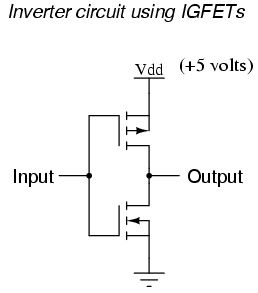Notice the "Vdd" label on the positive power supply terminal. This label follows the same convention as "Vcc" in TTL circuits: it stands for the constant voltage applied to the drain of a field effect transistor, in reference to ground.

Let's connect this gate circuit to a power source and input switch, and examine its operation. Please note that these IGFET transistors are E-type (Enhancement-mode), and so are normally-off devices. It takes an applied voltage between gate and drain (actually, between gate and substrate) of the correct polarity to bias them on.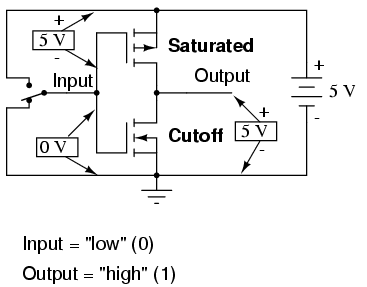The upper transistor is a P-channel IGFET. When the channel (substrate) is made more positive than the gate (gate negative in reference to the substrate), the channel is enhanced and current is allowed between source and drain. So, in the above illustration, the top transistor is turned on.

The lower transistor, having zero voltage between gate and substrate (source), is in its normal mode: off. Thus, the action of these two transistors are such that the output terminal of the gate circuit has a solid connection to Vdd and a very high resistance connection to ground. This makes the output "high" (1) for the "low" (0) state of the input.

Next, we'll move the input switch to its other position and see what happens: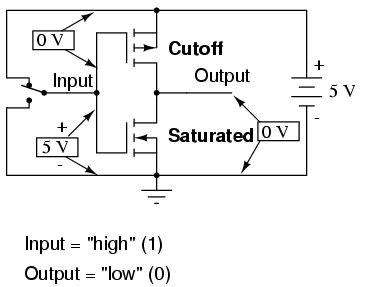Now the lower transistor (N-channel) is saturated because it has sufficient voltage of the correct polarity applied between gate and substrate (channel) to turn it on (positive on gate, negative on the channel). The upper transistor, having zero voltage applied between its gate and substrate, is in its normal mode: off. Thus, the output of this gate circuit is now "low" (0). Clearly, this circuit exhibits the behavior of an inverter, or NOT gate.

Using field-effect transistors instead of bipolar transistors has greatly simplified the design of the inverter gate. Note that the output of this gate never floats as is the case with the simplest TTL circuit: it has a natural "totem-pole" configuration, capable of both sourcing and sinking load current. Key to this gate circuit's elegant design is the complementary use of both P- and N-channel IGFETs. Since IGFETs are more commonly known as MOSFETs (Metal-Oxide-Semiconductor Field Effect Transistor), and this circuit uses both P- and N-channel transistors together, the general classification given to gate circuits like this one is CMOS: Complementary Metal Oxide Semiconductor.

CMOS circuits aren't plagued by the inherent nonlinearities of the field-effect transistors, because as digital circuits their transistors always operate in either the saturated or cutoff modes and never in the active mode. Their inputs are, however, sensitive to high voltages generated by electrostatic (static electricity) sources, and may even be activated into "high" (1) or "low" (0) states by spurious voltage sources if left floating. For this reason, it is inadvisable to allow a CMOS logic gate input to float under any circumstances. Please note that this is very different from the behavior of a TTL gate where a floating input was safely interpreted as a "high" (1) logic level.

This may cause a problem if the input to a CMOS logic gate is driven by a single-throw switch, where one state has the input solidly connected to either Vdd or ground and the other state has the input floating (not connected to anything):Also, this problem arises if a CMOS gate input is being driven by an open-collector TTL gate. Because such a TTL gate's output floats when it goes "high" (1), the CMOS gate input will be left in an uncertain state: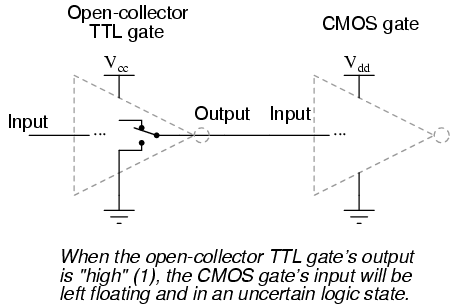Fortunately, there is an easy solution to this dilemma, one that is used frequently in CMOS logic circuitry. Whenever a single-throw switch (or any other sort of gate output incapable of both sourcing and sinking current) is being used to drive a CMOS input, a resistor connected to either Vdd or ground may be used to provide a stable logic level for the state in which the driving device's output is floating. This resistor's value is not critical: 10 kΩ is usually sufficient. When used to provide a "high" (1) logic level in the event of a floating signal source, this resistor is known as a pullup resistor: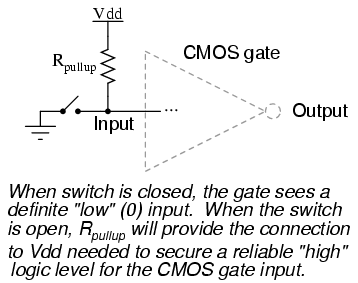When such a resistor is used to provide a "low" (0) logic level in the event of a floating signal source, it is known as a pulldown resistor. Again, the value for a pulldown resistor is not critical: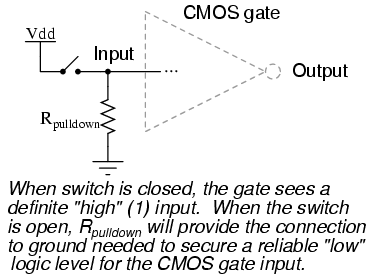Because open-collector TTL outputs always sink, never source, current, pullup resistors are necessary when interfacing such an output to a CMOS gate input: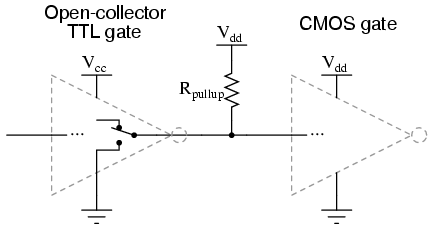Although the CMOS gates used in the preceding examples were all inverters (single-input), the same principle of pullup and pulldown resistors applies to multiple-input CMOS gates. Of course, a separate pullup or pulldown resistor will be required for each gate input: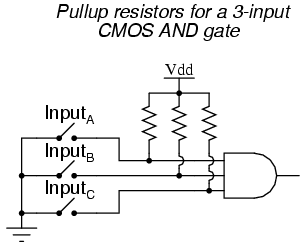This brings us to the next question: how do we design multiple-input CMOS gates such as AND, NAND, OR, and NOR? Not surprisingly, the answer(s) to this question reveal a simplicity of design much like that of the CMOS inverter over its TTL equivalent.

For example, here is the schematic diagram for a CMOS NAND gate: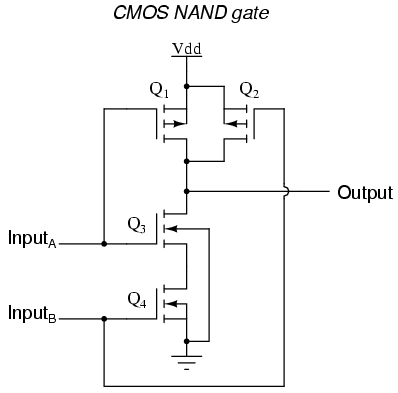Notice how transistors Q1 and Q3 resemble the series-connected complementary pair from the inverter circuit. Both are controlled by the same input signal (input A), the upper transistor turning off and the lower transistor turning on when the input is "high" (1), and vice versa. Notice also how transistors Q2 and Q4 are similarly controlled by the same input signal (input B), and how they will also exhibit the same on/off behavior for the same input logic levels. The upper transistors of both pairs (Q1 and Q2) have their source and drain terminals paralleled, while the lower transistors (Q3 and Q4) are series-connected. What this means is that the output will go "high" (1) if either top transistor saturates, and will go "low" (0) only if both lower transistors saturate. The following sequence of illustrations shows the behavior of this NAND gate for all four possibilities of input logic levels (00, 01, 10, and 11):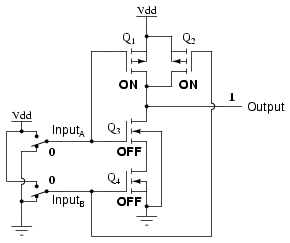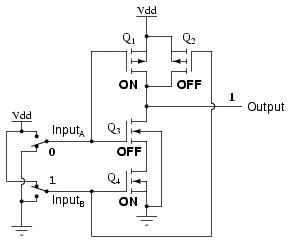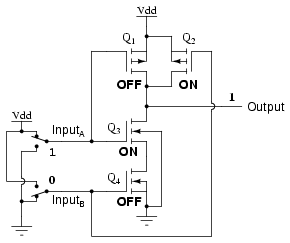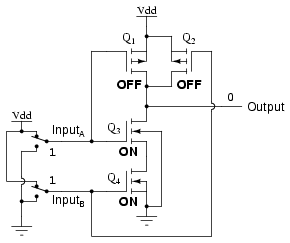As with the TTL NAND gate, the CMOS NAND gate circuit may be used as the starting point for the creation of an AND gate. All that needs to be added is another stage of transistors to invert the output signal: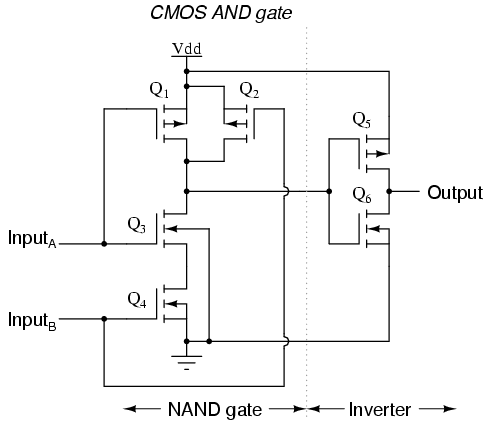A CMOS NOR gate circuit uses four MOSFETs just like the NAND gate, except that its transistors are differently arranged. Instead of two paralleled sourcing (upper) transistors connected to Vdd and two series-connected sinking (lower) transistors connected to ground, the NOR gate uses two series-connected sourcing transistors and two parallel-connected sinking transistors like this: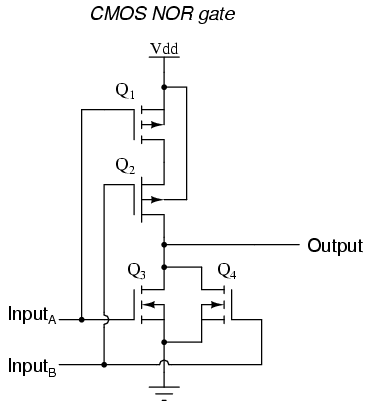As with the NAND gate, transistors Q1 and Q3 work as a complementary pair, as do transistors Q2 and Q4. Each pair is controlled by a single input signal. If either input A or input B are "high" (1), at least one of the lower transistors (Q3 or Q4) will be saturated, thus making the output "low" (0). Only in the event of both inputs being "low" (0) will both lower transistors be in cutoff mode and both upper transistors be saturated, the conditions necessary for the output to go "high" (1). This behavior, of course, defines the NOR logic function.

The OR function may be built up from the basic NOR gate with the addition of an inverter stage on the output: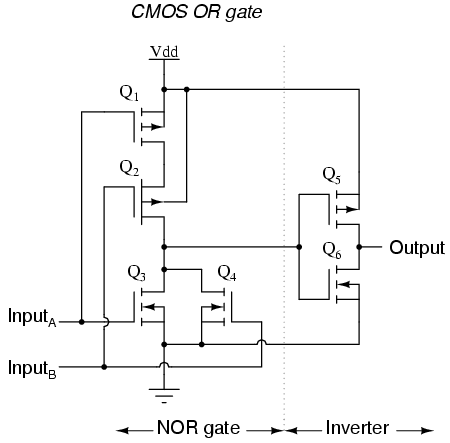Since it appears that any gate possible to construct using TTL technology can be duplicated in CMOS, why do these two "families" of logic design still coexist? The answer is that both TTL and CMOS have their own unique advantages.

First and foremost on the list of comparisons between TTL and CMOS is the issue of power consumption. In this measure of performance, CMOS is the unchallenged victor. Because the complementary P- and N-channel MOSFET pairs of a CMOS gate circuit are (ideally) never conducting at the same time, there is little or no current drawn by the circuit from the Vdd power supply except for what current is necessary to source current to a load. TTL, on the other hand, cannot function without some current drawn at all times, due to the biasing requirements of the bipolar transistors from which it is made.

There is a caveat to this advantage, though. While the power dissipation of a TTL gate remains rather constant regardless of its operating state(s), a CMOS gate dissipates more power as the frequency of its input signal(s) rises. If a CMOS gate is operated in a static (unchanging) condition, it dissipates zero power (ideally). However, CMOS gate circuits draw transient current during every output state switch from "low" to "high" and vice versa. So, the more often a CMOS gate switches modes, the more often it will draw current from the Vdd supply, hence greater power dissipation at greater frequencies.

A CMOS gate also draws much less current from a driving gate output than a TTL gate because MOSFETs are voltage-controlled, not current-controlled, devices. This means that one gate can drive many more CMOS inputs than TTL inputs. The measure of how many gate inputs a single gate output can drive is called fanout.

Another advantage that CMOS gate designs enjoy over TTL is a much wider allowable range of power supply voltages. Whereas TTL gates are restricted to power supply (Vcc) voltages between 4.75 and 5.25 volts, CMOS gates are typically able to operate on any voltage between 3 and 15 volts! The reason behind this disparity in power supply voltages is the respective bias requirements of MOSFET versus bipolar junction transistors. MOSFETs are controlled exclusively by gate voltage (with respect to substrate), whereas BJTs are current-controlled devices. TTL gate circuit resistances are precisely calculated for proper bias currents assuming a 5 volt regulated power supply. Any significant variations in that power supply voltage will result in the transistor bias currents being incorrect, which then results in unreliable (unpredictable) operation. The only effect that variations in power supply voltage have on a CMOS gate is the voltage definition of a "high" (1) state. For a CMOS gate operating at 15 volts of power supply voltage (Vdd), an input signal must be close to 15 volts in order to be considered "high" (1). The voltage threshold for a "low" (0) signal remains the same: near 0 volts.

One decided disadvantage of CMOS is slow speed, as compared to TTL. The input capacitances of a CMOS gate are much, much greater than that of a comparable TTL gate -- owing to the use of MOSFETs rather than BJTs -- and so a CMOS gate will be slower to respond to a signal transition (low-to-high or vice versa) than a TTL gate, all other factors being equal. The RC time constant formed by circuit resistances and the input capacitance of the gate tend to impede the fast rise- and fall-times of a digital logic level, thereby degrading high-frequency performance.

A strategy for minimizing this inherent disadvantage of CMOS gate circuitry is to "buffer" the output signal with additional transistor stages, to increase the overall voltage gain of the device. This provides a faster-transitioning output voltage (high-to-low or low-to-high) for an input voltage slowly changing from one logic state to another. Consider this example, of an "unbuffered" NOR gate versus a "buffered," or B-series, NOR gate: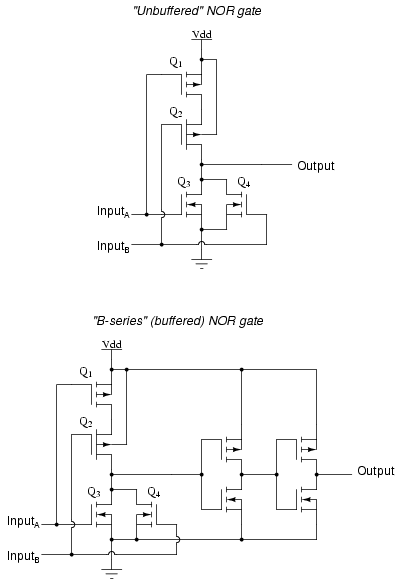In essence, the B-series design enhancement adds two inverters to the output of a simple NOR circuit. This serves no purpose as far as digital logic is concerned, since two cascaded inverters simply cancel: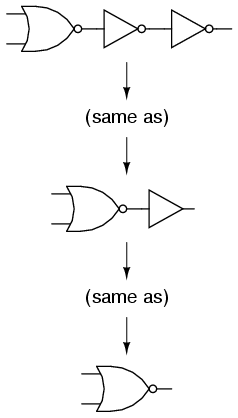However, adding these inverter stages to the circuit does serve the purpose of increasing overall voltage gain, making the output more sensitive to changes in input state, working to overcome the inherent slowness caused by CMOS gate input capacitance.

• REVIEW:
• CMOS logic gates are made of IGFET (MOSFET) transistors rather than bipolar junction transistors.
• CMOS gate inputs are sensitive to static electricity. They may be damaged by high voltages, and they may assume any logic level if left floating.
• Pullup and pulldown resistors are used to prevent a CMOS gate input from floating if being driven by a signal source capable only of sourcing or sinking current.
• CMOS gates dissipate far less power than equivalent TTL gates, but their power dissipation increases with signal frequency, whereas the power dissipation of a TTL gate is approximately constant over a wide range of operating conditions.
• CMOS gate inputs draw far less current than TTL inputs, because MOSFETs are voltage-controlled, not current-controlled, devices.
• CMOS gates are able to operate on a much wider range of power supply voltages than TTL: typically 3 to 15 volts versus 4.75 to 5.25 volts for TTL.
• CMOS gates tend to have a much lower maximum operating frequency than TTL gates due to input capacitances caused by the MOSFET gates.
• B-series CMOS gates have "buffered" outputs to increase voltage gain from input to output, resulting in faster output response to input signal changes. This helps overcome the inherent slowness of CMOS gates due to MOSFET input capacitance and the RC time constant thereby engendered.